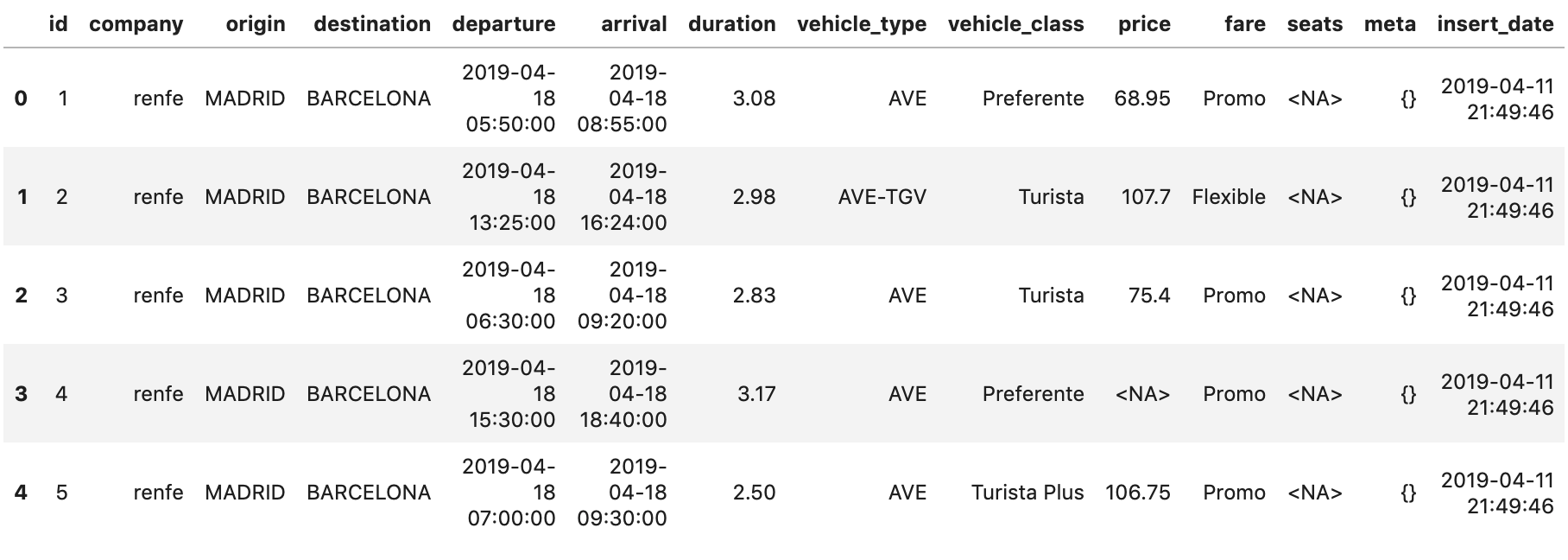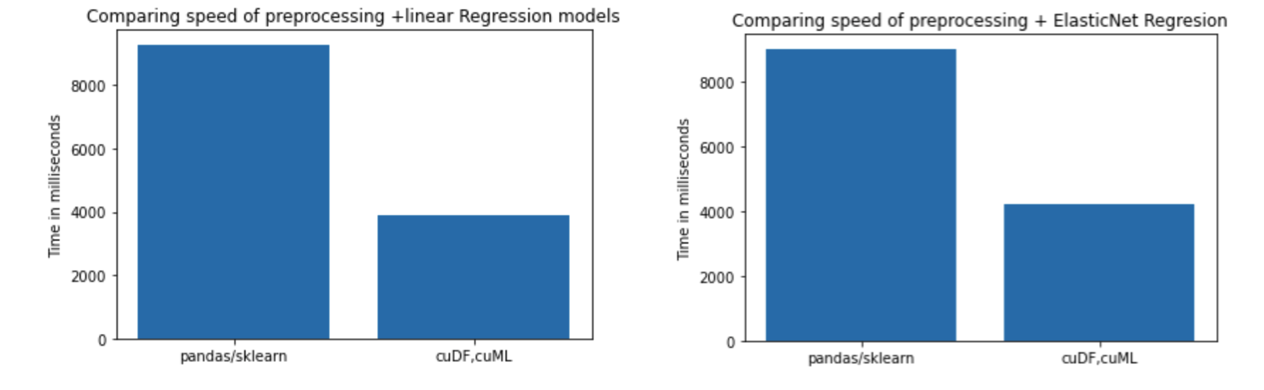# My First Experience Using RAPIDS

What I learned from using the GPU model training framework RAPIDS for the first time.If you’ve ever heard of RAPIDS, the tool from NVIDIA that uses GPUs for machine learning, you may have asked yourself: How tough is it to accelerate machine learning workflows? Will I need to take courses to learn this or watch some tutorials to understand scalability? Don’t worry, it turns out RAPIDS is very straightforward to learn.

One week earlier, I had similar questions about RAPIDS and after researching, I was surprised how simple it was to get started. I realized cuDF and cuML are two popular libraries of RAPIDS, whose API’s mirror those of pandas and sklearn.

If you know how to use pandas and sklearn for data preprocessing or training, you do not need to put any extra effort to make your model run faster. To chart out my journey with RAPIDS, I created 2 models: one that implements pandas/sklearn and other one with cuDF/cuML. In this article, I will go through each model step by step to scrutinize how with minimal changes, we can transition from pandas/sklearn to cuDF/cuML.

I am using Spanish Rail Ticket Pricing dataset from Kaggle, you can download data from their site.To escape the installation overhead of setting up RAPIDS, I will be using RAPIDS resource from Saturn Cloud which is a pre-build environment ready-made for running the tool.

## Using pandas/sklearn cuDF/cuML in parallel

First, I’ll walk through loading the data with the two methodologies. I read csv file using pandas.

``````import pandas as pd
``````

Instead of pandas, I imported cuDF. The read command is the same:

``````import cudf
``````

## Compare Data Engineering

Next, let’s compare how data engineering is different between the two platforms. Specifically, I have the objectives to:

1. Extract only the rows where column price is not null.
2. Convert the datatype for columns `departure`, `arrival` and `insert_date`.
3. Set column insert_date as index . Create new columns `Year`, `Hour`, `Month` from index.
4. Perform one hot coding over `origin` and `destination` so they are represented as binary data.

Below, is the code for implementation in pandas. You can see I used some basic commands; filtered the dataframe for rows which do not have missing price value and used `astype` function to change the data types from string to datetime. To set the dataframe index from column insert_date, I used method `set_index` and created new columns. Before starting with my last objective , I will select the variables which I will use for training. Dependent variables are `origin`, `destination`, `duration`, `hour`, `month` and `year` (X) and target variable is `price` (y). Now to convert categorical variables of X dataframe to binary vector form I pass columns through `get_dummies` .

#### Approach: Using pandas

``````#Extract only the rows where column price is not null .( Objective 1)
spain=spain[spain['price'].notnull()]

#Convert dataType for columns ‘departure’, ‘arrival' and   	'insert_date' ( Objective 2)
spain['departure'] = spain['departure'].astype('datetime64[ns]')
spain['arrival']=spain['arrival'].astype('datetime64[ns]')
spain['insert_date']=spain['insert_date'].astype('datetime64[ns]')

#Set column insert_date as index . Create new columns  ‘Year’,     	‘Hour’, ‘Month’ from index. ( Objective 3)
spain.set_index('insert_date',inplace = True)

#Create new columns ‘Year’, ‘Hour’, ‘Month’ from index.
spain['Year']=spain.index.year
spain['Hour']=spain.index.hour
spain['Month']=spain.index.month
#Splitting the data to dependent and independent variables
X=spain[['origin','destination','duration','Hour','Month','Year']]
y=spain['price']
#Perform one hot coding over ‘origin’ and ‘destination’ ( Objective 4)
X=pd.get_dummies(X, columns=['origin','destination'])
``````

Next, let’s see how to do it with cuDF. If you’ll notice, most of the commands are the exact same, such as changing the data type or filtering the dataframe. Sometimes they are slightly different, such as the one-hot encoding function name and parameters have changed. Refer to the cuDF API docs for more information on syntax .

#### Approach: Using cuDF

``````#Extract only the rows where column price is not null ( Objective 1)
spain=spain[spain['price'].notnull()]

#Convert dataType for columns ‘departure’, ‘arrival' and   	'insert_date' ( Objective 2)
spain['departure'] = spain['departure'].astype('datetime64[ns]')
spain['arrival']=spain['arrival'].astype('datetime64[ns]')
spain['insert_date']=spain['insert_date'].astype('datetime64[ns]')

#Set column insert_date as index . Create new columns  ‘Year’,     	‘Hour’, ‘Month’ from index. ( Objective 3)
spain.set_index('insert_date',inplace = True)

#Create new columns ‘Year’,‘Hour’, ‘Month’ from index.
spain['Year']=spain.index.year
spain['Hour']=spain.index.hour
spain['Month']=spain.index.month

#Splitting the data to dependent and independent variables
X=spain[['origin','destination','duration','Hour','Month','Year']]
y=spain['price']
#Perform one hot coding over ‘origin’ and ‘destination’ ( Objective 4)
X=X.drop(['destination','origin'],axis=1)

``````

## Compare Training

So far, I have played with data columns and compared pandas with cuDF. Verdict - cuDF seems easy to use. Most of the functions are the same as those of pandas. Let’s take the next step and see how the transition is from sklearn to cuML. I’m going to try using a linear regression in both platforms. I chose a linear regression because it is possibly the most simple common model in data science, and seemed like a straightforward comparison. That said, because it’s so simple, the benefit you get from using a linear regression on a GPU is less than, say, a random forest or more complex model being transitioned to a GPU.

Objective : Predict the price of train tickets given train’s origin, destination, duration, hour, month and year. I will first train my model using linear regression. Then I will go a step further and add regularization via ElasticNet Regression.

### Linear Regression

I start with importing `train_test_split` and `LinearRegression` from sklearn. Then calling the model, fitting the training set and predicting the test set.

#### Approach: Using sklearn

``````from sklearn.model_selection import train_test_split
from sklearn.linear_model import LinearRegression
x_train,x_test,y_train,y_test=train_test_split(X,y,test_size=0.2)
lr = LinearRegression(fit_intercept = True, normalize = False)
lr.fit(x_train,y_train)
preds = lr.predict(x_test)
``````

So, now that my linear regression model in sklearn is ready, let’s explore cuML. I’ll start by making changes to import statements. And that’s it! I did not change one bit for linear regression model. Verdict : It is so smooth to transition from skLearn to cuML. Don’t believe me? Let’s check the code below.

#### Approach: Using cuML

``````from cuml import train_test_split
from cuml import LinearRegression
x_train,x_test,y_train,y_test=train_test_split(X,y,test_size=0.2)
lr = LinearRegression(fit_intercept = True, normalize = False)
lr.fit(x_train,y_train)
preds = lr.predict(x_test)
``````

### ElasticNet Regression

The data scientist in me wants to regularize the above model by constraining weights and seeing how that works on the two different platforms. Hence, I choose ElasticNet, which is the combination of L1 and L2. Refer here for more information on Elastic Net.

#### Approach: Using sklearn

``````from sklearn.linear_model import ElasticNet
regr = ElasticNet(alpha = 0.1, l1_ratio=0.5)
result_enet=regr.fit(x_train,y_train)
preds = result_enet.predict(x_test)
``````

#### Approach: Using cuML

``````from cuml.linear_model import ElasticNet
regr = ElasticNet(alpha = 0.1, l1_ratio=0.5)
result_enet = regr.fit(x_train,y_train)
preds = result_enet.predict(x_test)
``````

Once again, all I did was replace the libraries! The plots below compare the execution times for pandas/sklearn, and cuDF/cuML. Clearly pandas/sklearn was outperformed by latter. For both the models, I have made my data engineering and training 2x faster. And while 2x faster is a good improvement, with a more complex data set or model type, the benefit can be far higher. This is because RAPIDS packages use GPU-based processing. We at Saturn Cloud have seen 2000x speed improvements by using GPUs and RAPIDS. In that example, we used multiple GPUs with RAPIDS and Dask together.### Wrapping Up

As a first time user of RAPIDS' cuDF and cuML libraries, I found it was easy to switch. If you are someone looking to increase the speed of their data processing and training with minimal changes to your existing code, look no further. If you are interested in trying RAPIDS yourself, join Saturn Cloud for free.

Image credit: SwapnIl Dwivedi

Try the code from this blog post in seconds on Saturn Cloud